## 8.4.2 Vector Fields Over Cell Complexes

This section describes how to construct a piecewise-smooth vector field over a cell complex. Only normalized vector fields will be considered. It is assumed that each cell in the complex has a simple shape over which it is easy to define a patch of the vector field. In many cases, the cell decomposition techniques that were introduced in Chapter 6 for motion planning can be applied to construct a feedback plan.

Suppose that an-dimensional state spacehas been decomposed into a cell complex, such as a simplicial complex or singular complex, as defined in Section 6.3.1. Assume that the goal set is a single point,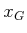. Defining a feedback plan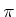overrequires placing a vector field onfor which all integral curves lead to(ifis reachable). This is accomplished by defining a smooth vector field for each-cell. Each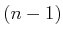-cell is a switching boundary, as considered in Section 8.3.1. This leads directly to solution trajectories in the sense of Filipov. Ifis allowed to be discontinuous, then it is actually not important to specify values on any of the cells of dimension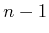or less.

A hierarchical approach is taken to the construction of:

1. Define a discrete planning problem over the-cells. The cell that containsis designated as the goal, and a discrete navigation function is defined over the cells.
2. Define a vector field over each-cell. The field should cause all states in the cell to flow into the next cell as prescribed by the discrete navigation function.
One additional consideration that is important in applications is to try to reduce the effect of the discontinuity across the boundary as much as possible. It may be possible to eliminate the discontinuity, or even construct a smooth transition between-cells. This issue will not be considered here, but it is nevertheless quite important [235,643].

The approach will now be formalized. Suppose that a cell complex has been defined over a continuous state space,. Let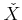denote the set of-cells, which can be interpreted as a finite state space. A discrete planning problem will be defined over. To avoid confusion with the original continuous problem, the prefix super will be applied to the discrete planning components. Each superstate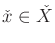corresponds to an-cell. From each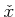, a superaction,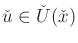exists for each neighboring-cell (to be neighboring, the two cells must share an-dimensional boundary). Let the goal superstate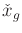be the-cell that contains. Assume that the cost functional is defined for the discrete problem so that every action (other than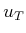) produces a unit cost. Now the concepts from Section 8.2 can be applied to the discrete problem. A discrete navigation function,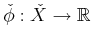, can be computed using Dijkstra's algorithm (or another algorithm, particularly if optimality is not important). Using the discrete local operator from Section 8.2.2, this results in a discrete feedback plan,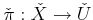.

Based on the discrete feedback plan, there are two kinds of-cells. The first is the goal cell,, for which a vector field needs to be defined so that all integral curves lead to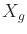in finite time.8.11 A termination action can be applied whenis actually reached. The remaining-cells are of the second kind. For each cell, the boundary that is shared with the cell reached by applying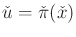is called the exit face. The vector field over the-cellmust be defined so that all integral curves lead to the exit face. When the exit face is reached, a transition will occur into the next-cell. If the-cells are convex, then defining this transition is straightforward (unless there are additional requirements on the field, such as smoothness at the boundary). For more complicated cells, one possibility is to define a vector field that retracts all points onto a single curve in the cell.

A simple example of the approach is illustrated for the case of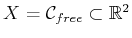, in which the boundary ofis polygonal. This motion planning problem was considered in Section 6.2, but without feedback. Suppose that a triangulation ofhas been computed, as described in Section 6.3.2. An example was shown in Figure 6.16. A discrete feedback plan is shown for a particular goal state in Figure 8.10. Each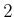-cell (triangle) is labeled with an arrow that points to the next cell.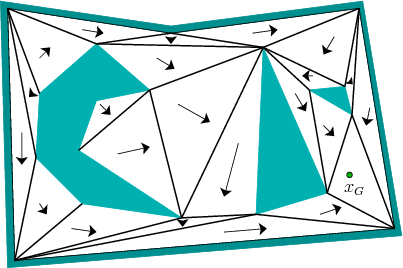For the cell that contains, a normalized version of the inward vector field shown in Figure 8.5b can be formed by dividing each nonzero vector by its magnitude. It can then be translated to move its origin to. For each remaining-cell, a vector field must be constructed that flows into the appropriate neighboring cell. Figure 8.11 illustrates a simple way to achieve this. An outward vector field can be made by negating the field shown in Figure 8.5b to obtain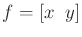. This field can be normalized and translated to move the origin to the triangle vertex that is not incident to the exit edge. This is called the repulsive vertex in Figure 8.11. This generates a vector field that pushes all points in the triangle to the ext edge. If the fields are constructed in this way for each triangle, then the global vector field represents a solution feedback plan for the problem. Integral curves (in the sense of Filipov) lead toin finite time.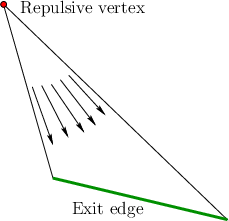Steven M LaValle 2020-08-14